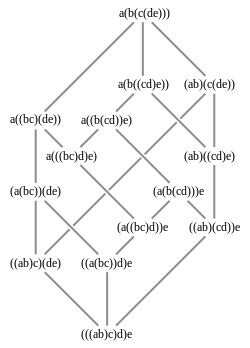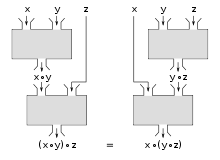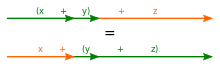# Associative property

"Associative" and "non-associative" redirect here. For associative and non-associative learning, see Learning § Types.

In mathematics, the associative property is a property of some binary operations. In propositional logic, associativity is a valid rule of replacement for expressions in logical proofs.

Within an expression containing two or more occurrences in a row of the same associative operator, the order in which the operations are performed does not matter as long as the sequence of the operands is not changed. That is, rearranging the parentheses in such an expression will not change its value. Consider the following equations:Even though the parentheses were rearranged on each line, the values of the expressions were not altered. Since this holds true when performing addition and multiplication on any real numbers, it can be said that "addition and multiplication of real numbers are associative operations".

Associativity is not to be confused with commutativity, which addresses whether or not the order of two operands changes the result. For example, the order doesn't matter in the multiplication of real numbers, that is, a × b = b × a, so we say that the multiplication of real numbers is a commutative operation.

Associative operations are abundant in mathematics; in fact, many algebraic structures (such as semigroups and categories) explicitly require their binary operations to be associative.

However, many important and interesting operations are non-associative; some examples include subtraction, exponentiation and the vector cross product. In contrast to the theoretical counterpart, the addition of floating point numbers in computer science is not associative, and is an important source of rounding error.

## DefinitionA binary operation ∗ on the set S is associative when this diagram commutes. That is, when the two paths from S×S×S to S compose to the same function from S×S×S to S.

Formally, a binary operation ∗ on a set S is called associative if it satisfies the associative law:

(xy) ∗ z = x ∗ (yz) for all x, y, z in S.

Here, ∗ is used to replace the symbol of the operation, which may be any symbol, and even the absence of symbol (juxtaposition) as for multiplication.

(xy)z = x(yz) = xyz for all x, y, z in S.

The associative law can also be expressed in functional notation thus: f(f(x, y), z) = f(x, f(y, z)).

## Generalized associative lawIn the absence of the associative property, five factors a, b, c, d, e result in a Tamari lattice of order four, possibly different products.

If a binary operation is associative, repeated application of the operation produces the same result regardless how valid pairs of parenthesis are inserted in the expression. This is called the generalized associative law. For instance, a product of four elements may be written in five possible ways:

1. ((ab)c)d
2. (ab)(cd)
3. (a(bc))d
4. a((bc)d)
5. a(b(cd))

If the product operation is associative, the generalized associative law says that all these formulas will yield the same result, making the parenthesis unnecessary. Thus "the" product can be written unambiguously as

abcd.

As the number of elements increases, the number of possible ways to insert parentheses grows quickly, but they remain unnecessary for disambiguation.

## ExamplesIn associative operations is.The addition of real numbers is associative.

Some examples of associative operations include the following.

• The concatenation of the three strings "hello", " ", "world" can be computed by concatenating the first two strings (giving "hello ") and appending the third string ("world"), or by joining the second and third string (giving " world") and concatenating the first string ("hello") with the result. The two methods produce the same result; string concatenation is associative (but not commutative).
• In arithmetic, addition and multiplication of real numbers are associative; i.e.,Because of associativity, the grouping parentheses can be omitted without ambiguity.• If M is some set and S denotes the set of all functions from M to M, then the operation of functional composition on S is associative:• Slightly more generally, given four sets M, N, P and Q, with h: M to N, g: N to P, and f: P to Q, thenas before. In short, composition of maps is always associative.
• Consider a set with three elements, A, B, and C. The following operation:
× A B C
A A A A
B A B C
C A A A
is associative. Thus, for example, A(BC)=(AB)C = A. This operation is not commutative.

## Propositional logic

### Rule of replacement

In standard truth-functional propositional logic, association, or associativity are two valid rules of replacement. The rules allow one to move parentheses in logical expressions in logical proofs. The rules are:andwhere "" is a metalogical symbol representing "can be replaced in a proof with."

### Truth functional connectives

Associativity is a property of some logical connectives of truth-functional propositional logic. The following logical equivalences demonstrate that associativity is a property of particular connectives. The following are truth-functional tautologies.

Associativity of disjunction:Associativity of conjunction:Associativity of equivalence:## Non-associativity

A binary operationon a set S that does not satisfy the associative law is called non-associative. Symbolically,For such an operation the order of evaluation does matter. For example:Also note that infinite sums are not generally associative, for example:whereasThe study of non-associative structures arises from reasons somewhat different from the mainstream of classical algebra. One area within non-associative algebra that has grown very large is that of Lie algebras. There the associative law is replaced by the Jacobi identity. Lie algebras abstract the essential nature of infinitesimal transformations, and have become ubiquitous in mathematics.

There are other specific types of non-associative structures that have been studied in depth; these tend to come from some specific applications or areas such as combinatorial mathematics. Other examples are Quasigroup, Quasifield, Non-associative ring, Non-associative algebra and Commutative non-associative magmas.

### Nonassociativity of floating point calculation

In mathematics, addition and multiplication of real numbers is associative. By contrast, in computer science, the addition and multiplication of floating point numbers is not associative, as rounding errors are introduced when dissimilar-sized values are joined together.

To illustrate this, consider a floating point representation with a 4-bit mantissa:
(1.0002×20 + 1.0002×20) + 1.0002×24 = 1.0002×21 + 1.0002×24 = 1.0012×24
1.0002×20 + (1.0002×20 + 1.0002×24) = 1.0002×20 + 1.0002×24 = 1.0002×24

Even though most computers compute with a 24 or 53 bits of mantissa, this is an important source of rounding error, and approaches such as the Kahan Summation Algorithm are ways to minimise the errors. It can be especially problematic in parallel computing.

### Notation for non-associative operations

In general, parentheses must be used to indicate the order of evaluation if a non-associative operation appears more than once in an expression. However, mathematicians agree on a particular order of evaluation for several common non-associative operations. This is simply a notational convention to avoid parentheses.

A left-associative operation is a non-associative operation that is conventionally evaluated from left to right, i.e.,while a right-associative operation is conventionally evaluated from right to left:Both left-associative and right-associative operations occur. Left-associative operations include the following:

• Subtraction and division of real numbers:• Function application:This notation can be motivated by the currying isomorphism.

Right-associative operations include the following:One reason exponentiation is right-associative is that a repeated left-associative exponentiation operation would be less useful. Multiple appearances could (and would) be rewritten with multiplication:An additional argument for exponentiation being right-associative is that the superscript inherently behaves as a set of parentheses; e.g. in the expressionthe addition is performed before the exponentiation despite there being no explicit parentheseswrapped around it. Thus given an expression such as, it makes sense to require evaluating the full exponentof the basefirst.Using right-associative notation for these operations can be motivated by the Curry-Howard correspondence and by the currying isomorphism.

Non-associative operations for which no conventional evaluation order is defined include the following.• Taking the pairwise average of real numbers:• Taking the relative complement of setsis not the same as. (Compare material nonimplication in logic.)Look up associative property in Wiktionary, the free dictionary.

## References

1. Hungerford, Thomas W. (1974). Algebra (1st ed.). Springer. p. 24. ISBN 0387905189. Definition 1.1 (i) a(bc) = (ab)c for all a, b, c in G.
2. Durbin, John R. (1992). Modern Algebra: an Introduction (3rd ed.). New York: Wiley. p. 78. ISBN 0-471-51001-7. Ifare elements of a set with an associative operation, then the productis unambiguous; this is, the same element will be obtained regardless of how parentheses are inserted in the product
3. "Matrix product associativity". Khan Academy. Retrieved 5 June 2016.
4. Moore and Parker
5. Copi and Cohen
6. Hurley
7. Knuth, Donald, The Art of Computer Programming, Volume 3, section 4.2.2
8. IEEE Computer Society (August 29, 2008). "IEEE Standard for Floating-Point Arithmetic". IEEE. doi:10.1109/IEEESTD.2008.4610935. ISBN 978-0-7381-5753-5. IEEE Std 754-2008.
9. Villa, Oreste; Chavarría-mir, Daniel; Gurumoorthi, Vidhya; Márquez, Andrés; Krishnamoorthy, Sriram, Effects of Floating-Point non-Associativity on Numerical Computations on Massively Multithreaded Systems (PDF), retrieved 2014-04-08
10. Goldberg, David (March 1991). "What Every Computer Scientist Should Know About Floating-Point Arithmetic" (PDF). ACM Computing Surveys. 23 (1): 5–48. doi:10.1145/103162.103163. Retrieved 2016-01-20. (, )